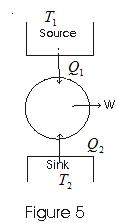# Heat Engine and efficiency

## Heat Engine and efficiency

• Any device which convents heat continuously into mechanical work is called a heat engine.
• For any heat engine there are three essential requirements.
(i) SOURCE : A hot body at fixed temperature T1 from which heat engine can draw heat
(ii) Sink : A cold body, at a fixed lower temperature T2, to which any amount of heat can be rejected.
(iii) WORKING SUBTANCE : The material, which on being supplied with heat will do mechanical work.
• In heat engine, working substances, could be gas in cylinder with a moving piston.
• In heat engine working substance takes heat from the source, convents a part of it into mechanical work, gives out rest to the sink and returns to the initial state. This series of operations constitutes a cycle.
• This cycle is represented in fig below• Work from heat engine can be continuously obtained by performing same cycle again and again.
• Consider,
$Q_1$ - heat absorbed by working substance from source
$Q_2$ - heat rejected to the since
$W$ - net amount of work done by working substance
$Q_1-Q_2$ - net amount of heat absorbed by working substance.
$\Delta U = 0$ since in the cycle Working Substance returns to its initial condition.
So on application of first law of thermodynamics
$Q_1- Q_2 = W$
• Thermal efficiency of heat engine
$\eta = \frac {\text {work output in energy units}}{ \text {Heat input in same energy units}}$
$\eta = \frac {W }{ Q_1} = \frac {Q_1-Q_2}{ Q_1}$
Or, $\eta = 1- \frac {Q_2}{Q_1}$ from this equation it is clear that
$\eta = 1$ for Q2=0
and there would be 100% conversion of heat absorbed into work but such ideal engines are not possible in practice.